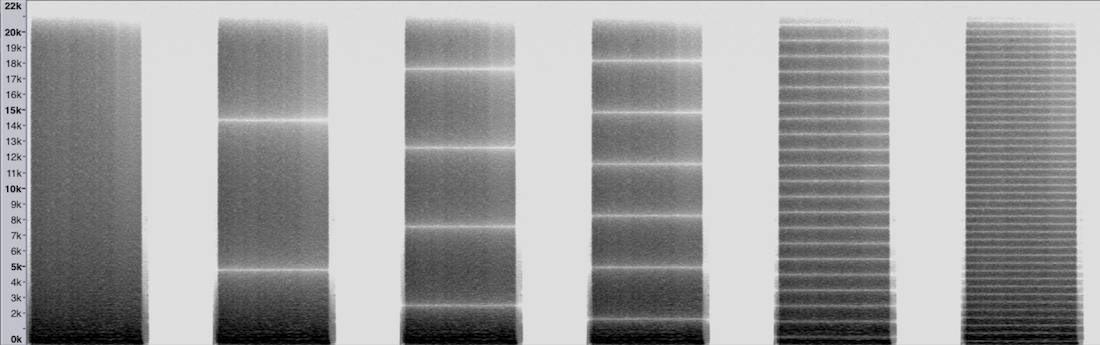# Comb filter example

When an identical copy of a waveform is time-shifted (by small amounts) and added to the original, comb filtering occurs. This means that there is cancelation between the original and shifted waveforms at distinct frequencies.

Here is an example.

The original sample is a short chord played on an electric guitar through a distortion pedal (examples of this phenomena are best with a large bandwidth sounds).

The sound is played once, then the sound is played several times with a shifted copy of the sound played with it.

The shifts are 0.0001, 0.0002, 0.0003, 0.001, and 0.002 seconds.

Here is a spectrogram of the sound file. You can nicely see the comb filter as sets of equi-spaced light horizontal lines through each repeated copy. Each line corresponds to a different frequency at which cancelation has occurred.Why does this cancelling happen?

First, imagine a sine wave.

Suppose it has period (i.e., wavelength) P.

If we create another sine wave that is a shifted copy of this sine wave, and the shift is an odd multiple of P/2, then the sum of the two waves will be zero. (For now, convince yourself that this is true by drawing some pictures).

This gives us the very simple equation S = n (P/2) where S is the amount of the shift and n is an odd integer.

Then P = 2S/n and so the frequency of the waveform, 1/P, is
1/P = n/(2S)
that is,
1/P = n (1/(2S)).

So for example, if the shift is S=0.0002 seconds, then the frequencies where we cancel are given by
n (1/(2*0.0002)) = 2500 n,
in other words, all odd multiples of 2500 hertz. This is what we see in the spectrogram: white lines indicating cancelation at 2500 hz, 7500 hz, 12500 hz and 17500 hz (these are in the third segment from the left in the image above).